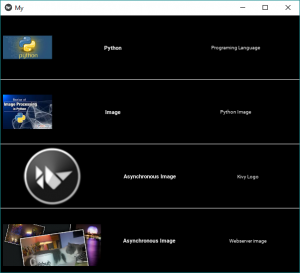Open In App
Related Articles
• Write an Interview Experience
• Kivy Tutorial

# Python | Adding image in Kivy using .kv file

Kivy is a platform independent GUI tool in Python. As it can be run on Android, IOS, linux, and Windows, etc. It is basically used to develop the Android application, but it does not mean that it can not be used on Desktops applications.

### Image Widget:

The Image widget is used to display an image. To use the image widget you must have to import :

from kivy.uix.image import Image, AsyncImage (not necessary while working with .kv file)

because the module kivy.uix.image have all the functionality related to images.
Images can be loaded to the Application via two types:

Note: By default, the image is centered and fits inside the widget bounding box. If you don’t want that, you can set allow_stretch to True and keep_ratio to False.

```Basic Approach to create multiple layout in one file:
1) import kivy
2) import kivyApp
3) import image
4) import BoxLayout
5) set minimum version(optional)
6) Create the Layout class
7) Create App class
8) Create .kv file:
4) Resizing, Positioning etc of Image
9) return instance of the layout class
10) Run an instance of the class```

So in the below code, we will explain How to load Synchronous and Asynchronous images. Also How to resize, Positioning, Label, etc the image with some more stuff.
.py file –

## Python3

 `## Sample Python application demonstrating the ``## working with images in Kivy using .kv file ``  ` `##################################################``# import kivy module   ``import` `kivy ``    ` `# base Class of your App inherits from the App class.   ``# app:always refers to the instance of your application  ``from` `kivy.app ``import` `App``  ` `# this restrict the kivy version i.e ``# below this kivy version you cannot ``# use the app or software ``kivy.require(``'1.9.0'``)` `# BoxLayout arranges children in a vertical or horizontal box.``# or help to put the children at the desired location.``from` `kivy.uix.boxlayout ``import` `BoxLayout`` ` `# to change the kivy default settings we use this module config``from` `kivy.config ``import` `Config`` ` `# 0 being off 1 being on as in true / false``# you can use 0 or 1 && True or False``Config.``set``(``'graphics'``, ``'resizable'``, ``True``)` `# creating the root widget used in .kv file``class` `Imagekv(BoxLayout):``    ``'''``        ``no need to do anything here as``        ``we are building things in .kv file``    ``'''``    ``pass` `# class in which name .kv file must be named My.kv.``class` `MyApp(App):``    ``# define build() function``    ``def` `build(``self``):``        ``# returning the instance of Imagekv class``        ``return` `Imagekv()` `# run the App``if` `__name__ ``=``=` `'__main__'``:``    ``MyApp().run()`

.kv file implementation –

## Python3

 `# How to use images in kivy using .kv` `# root widget of Imagekv Class``:` `    ``# Giving orientation to Box Layout``    ``orientation:``'vertical'` `###############################################`` ` `    ``# Adding Box Layout``    ``BoxLayout:``        ` `        ``padding:``5` `        ``# Adding image from the system``        ``Image:``            ``source: ``'download.jpg'` `            ``# Giving the size of image``            ``size_hint_x: ``0.4` `            ``# allow stretching of image``            ``allow_stretch: ``True``            ` `        ``# Giving Label to images``        ``Label:``            ``text:``"Python"``            ``font_size:``11``            ``bold:``True` `        ``Label:``            ``text:``"Programming Language"``            ``font_size:``10``            ` `###############################################`` ` `   ``# Drawing the line between the multiples``    ``Label:``        ``canvas.before:``            ``Color:``                ``rgba: (``1``, ``1``, ``1``, ``1``)``            ``Rectangle:``                ``size: ``self``.size``                ``pos: ``self``.pos``        ``size_hint_y: ``None``        ``height: ``1` `################################################`` ` `    ``# Another Box Layout``    ``BoxLayout:``        ``padding:``5``        ``Image:``            ``source:``"downloading.jpg"``            ``size_hint_x: ``0.4``            ``allow_stretch: ``True` `        ``Label:``            ``text:``"Image"``            ``font_size:``11``            ``bold:``True` `        ``Label:``            ``text:``"Python Image"``            ``font_size:``10``            ` `#############################################`` ` `    ``# Drawing the line between the multiples``    ``Label:``        ``canvas.before:``            ``Color:``                ``rgba: (``1``, ``1``, ``1``, ``1``)``            ``Rectangle:``                ``size: ``self``.size``                ``pos: ``self``.pos``        ``size_hint_y: ``None``        ``height: ``1``        ` `###############################################`` ` `    ``# Adding next Box Layout``    ``BoxLayout:``        ``padding:``5` `        ``# To load an image asynchronously``        ``# (for example from an external webserver)``        ``AsyncImage:``            ``source: ``'http://kivy.org/logos/kivy-logo-black-64.png'``            ``width: ``100``            ``allow_stretch: ``True` `        ``Label:``            ``text:``" Asynchronous Image "``            ``font_size:``11``            ``bold:``True` `        ``Label:``            ``text:``"Kivy Logo"``            ``font_size:``10` `####################################################``            ` `    ``# Drawing the line between the multiples``    ``Label:``        ``canvas.before:``            ``Color:``                ``rgba: (``1``, ``1``, ``1``, ``1``)``            ``Rectangle:``                ``size: ``self``.size``                ``pos: ``self``.pos``        ``size_hint_y: ``None``        ``height: ``1` `#####################################################`` ` `    ``# LAst Box Layout``    ``BoxLayout:``        ``padding:``5``        ` `        ``AsyncImage:``            ``size_hint_y: ``None``            ``source: ``'http://kivy.org/slides/kivypictures-thumb.jpg'``            ``width: ``100``            ``allow_stretch: ``True` `        ``Label:``            ``text:``"Asynchronous Image "``            ``font_size:``11``            ``bold:``True` `        ``Label:``            ``text:``" Webserver image "``            ``font_size:``10`

Output: